## Year 4 maths worksheet: More addition and subtraction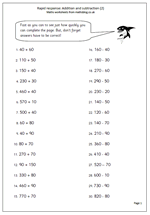Here is a second page of questions on adding and subtracting multiples of 10, which year 4 children should be able to take on and answer rapidly.

It is always fascinating to ask children how they work things out in their heads. Let’s look at 330 + 90.  I would do this by adding 100 to make 430 and then subtracting 10 to make 420. It could also be done by adding 33 and 9 to make 42 and adjusting to take account of the hundreds; and there are other ways. Any way which reaches a correct answer is OK, but we are really looking for methods which are very fast and if children are not using them they should be shown.

Rapid response: Add and subtract (2)

## Resource of the Week: Written methods of subtraction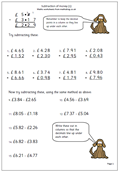This week we are highlighting in our series of worksheets covering the four rules, especially written methods of subtraction. Children should be competent with the standard written method of subtraction before moving onto subtracting decimals. Money is an excellent way to show how decimals work and it is important to ensure that there are two numbers after the decimal point. Another key point, of course, is to make sure the columns are correctly in line and that a decimal point is included in the answer. Make sure that questions 9 to 15 are written out in the correct format: vertically rather than horizontally.

Standard money subtraction (p1)

## Year 3 maths worksheet: division by hopping along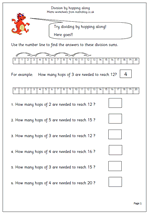A neat way to show division is to use a number line and ‘hop’ along in equal steps of whatever the number is you are dividing by.  There are no remainders to the answers on this page, but it is also a good way to show why remainders occur.

This is an excellent page for those children in Year 3 who find the idea of division difficult, as it also gives a clue to the relationship between division and multiplication. This page can be found as part of our year 3 calculating worksheets.

Divide by hopping

## The Little Maths Book: an intro to algebra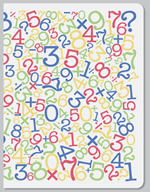Beginning algebra can be tricky – and that’s where The Little Maths Book comes in. The idea is to move away from the usual dull, functional explanations and come up with something small and beautiful which is a great introduction to algebra.
Two brothers (a maths tutor and award winning graphic designer) have got together to produce a clever little book concentrating on the fundamental ideas of algebra, but with engaging graphics of birds, balloons, buses etc. It has clever fold out pages which show visually what happens when you balance an equation, almost like a pop-up book.
Aimed at any age, this is a nifty book which makes understanding algebra interesting and fun for both young and old alike. Certainly an excellent introduction for year 7 students.

The Little Algebra Book

## Year 6 maths worksheet: Division with decimals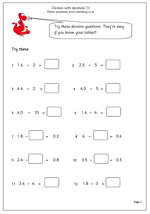Once children have a really good knowledge of ‘tables’ and understand place value they can apply this knowledge in all sorts of ways. One way is to mentally calculate division with decimals.

16 divided by 2 is easy: 8

1.6 divided by 2 can cause all sorts of problems, but, of course is 0.8.

When I try to do a question such as 2.5 divided by 5 I mentally calculate 25 divided by 5 and then divide the answer by 10 ‘in my head’ by moving each number one place to the right. There are other ways but this seems quite efficient.

It is harder when faced with a question such as 1.8 divided by what makes 0.2? Again, if both numbers are multiplied by 10 it becomes 18 divided by what makes 2, which is easy: 9.

This Year 6 maths worksheet on division with decimals is a good test of how well children can manipulate numbers and use their knowledge.

Divide with decimals (1)

## Coming soon: Division, decimals and mental arithmeticNext week we will have a Year 6 maths worksheet on division with decimals, which is a good test of how well children can manipulate numbers and use their knowledge.

16 divided by 2 is easy: 8

1.6 divided by 2 can cause all sorts of problems, but, of course is 0.8.

When I try to do a question such as 2.5 divided by 5 I mentally calculate 25 divided by 5 and then divide the answer by 10 ‘in my head’ by moving each number one place to the right. There are other ways but this seems quite efficient.

For younger children a neat way to show division is to use a number line and ‘hop along’ in equal steps of whatever the number is you are dividing by.  There are no remainders to the answers on this forthcoming page, but it is also a good way to show why remainders occur if there are not enough numbers to ‘hop along’.

We will also be publishing a second page of questions on adding and subtracting multiples of 10, which year 4 children should be able to take on and answer rapidly.

## Year 3 maths worksheet: dividing by 1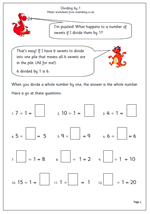Dividing by one might seem a bit obvious to us, but it is not always the case for children who don’t yet have a good understanding of division.

Dividing by 1 can be explained in two ways:

sharing equally – the sweets are shared equally between 1 person, in other words the lucky person gets the whole lot!

grouping – there are 6 sweets to be put into 1 group.

Sometimes even adults become confused with dividing by one, and the more complex idea of dividing by zero (how many zeros are there in 10 whole ones????)!

This is probably most suited to Year 3 children.

Divide by 1

## Resource of the Week: Times tables and square numbers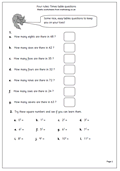As well as all our worksheets in each of the year groups from 1 to 6 don’t forget to look at our Four Rules section for the more ‘old fashioned’ type of pages. Here we have rather a mixed bag of questions. The first half asks questions derived from the times tables, such as ‘How many fours in 32?’ This is an important question to ask before moving on to the long method of division.

The second set of questions looks at square numbers, which, of course, give a diagonal pattern on a tables square. Children need to be familiar with the square sign to tackle this. It is extremely useful to know, off by heart, all the square numbers, up to at least 10 x 10, as it will be a great help later in High School.

This page can be found in our Four Rules section, under Multiplication (mental methods).

Times table questions (pg 1)

## Year 4 maths worksheet: Dividing by 10 and 100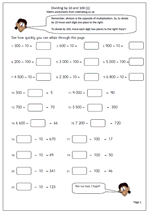Children generally find division harder than multiplication. This is often because the process involves multiplying to find the answer, especially with pencil and paper methods. This page, however,  is aimed at rapid answering using mental methods.

Dividing by ten is the inverse of multiplying by ten. To multiply by 10 move each digit one place to the left. To divide by ten move each digit one place to the right.

Because all the numbers are multiples of 10 there is no need to introduce the decimal point at this stage.

To divide by 100 move two places to the right.

The later questions have different parts of the number sentence to complete: multiplying is the easiest way to do these.

Divide by 10 and 100 (1)

## Year 6 maths worksheet: Factors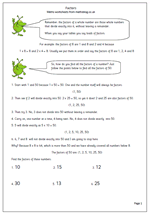Understanding factors and multiples is something many children fail to grasp. Here we have a page on factors and how to find all the factors of a number. The process is fairly straightforward, but can be quite time consuming. A good knowledge of tables and division is also needed. So if children’s knowledge of tables is weak, if they find division difficult and if they have little staying power then it is unlikely that they will enjoy trying to find factors of numbers!

One point that even brighter children take a while to understand is that you only need to continue to divide up to when the number squared is larger than the original number eg to find the factors of 62 you only need to divide 62 by numbers up to 7 because eight eights are 64. It is a good idea to spend some time explaining and showing the logic of this to children.

This can be found in our Year 6 Understanding Number section.

Factors 1